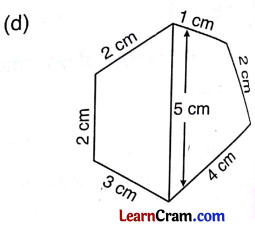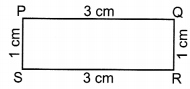# DAV Class 4 Maths Chapter 11 Worksheet 2 Solutions

The DAV Maths Book Class 4 Solutions and DAV Class 4 Maths Chapter 11 Worksheet 2 Solutions of Perimeter offer comprehensive answers to textbook questions.

## DAV Class 4 Maths Ch 11 WS 2 Solutions

Question 1.
Find the perimeter of the following figures by measuring the sides.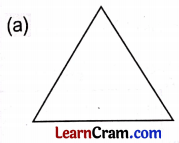Perimeter = sum of all sides,
perimeter of Δ = 3.5 + 3.8 + 3.5
= 10.8 cm.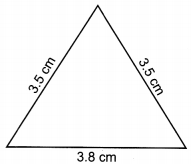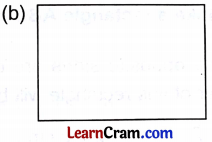Perimeter = sum of all sides
= 3 + 4.4 + 3 + 4.4
= 14.8 cm.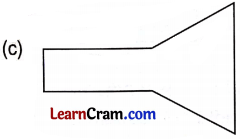Perimeter = sum of all sides.
= 0.8 + 3 + 3 + 2.2 + 2.2 + 2.7
= 11.2 cm.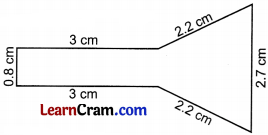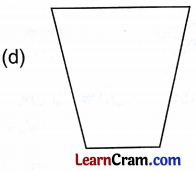Perimeter = sum of all sides
= 3.5 + 3.5 + 3.5 + 1.8
= 13.9 cm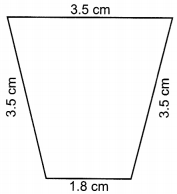Question 2.
Find the perimeters of the following figures using the measurements given below.Perimeter of figure
= 4 + 3 + 4 + 3
= 14 cm.Perimeter of figure
= 2 + 2 + 4 + 4 + 3
= 15 cmPerimeter of figure
= 1 + 4 + 4+ 1 + 3 + 3
= 16 cm